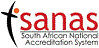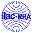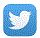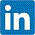## Useful information:

Concentration units | Weight or mass units | Length units | Liquid or volume units | SI prefixes | Recipe or cooking conversions | Temperature scales | Rate of Flow | Acid factors | Food Energy

### Concentration units:

• parts per million = ppm = 1 part solute in 1 000 000 parts solution
• 1 microgram/liter (ug/l) = 1 part per billion (ppb)
• 1 ppm by volume = 1 mg/l = 1 microgram/ml = 1 ng/microliter = 1 % m/v x 0.0001
• 1 ppm by weight = 1 mg/kg = 1 microgram/g = 1 ng/mg = 1 % m/m x 0.0001
• 1% = 1 part solute per 100 parts solution
• 1% m/v= 1 mass part solute per 100 volume parts solution
• 1% m/m= 1 mass part solute per 100 mass parts solution
• 10% = 10 parts solute per 100 parts solution
• 1 gram/tonne (g/T) = 1 part per million (ppm) = 1 milligram/liter (mg/l)

### Weight or mass units (approximate):

• gram = gramme = g = gm but g also is for gravity, where g = 9.8 m/s2 = 32.2 ft/s2
• 1 kilogram (kg) = 1000 grams (g)
• 1 gram (g or gm) = 1000 milligrams (mg)
• 1 milligram (mg) = 1000 micrograms (µg)
• 1 microgram (µg or mcg) = 1000 nanograms (ng)
• 1 nanogram (ng) = 1000 picograms (pg)
• 1 metric carat = 200mg
• 1 gram = 15 grains Apothecary
• 1 dram = 4 gram = 60 grains Apothecary
• 1 grain = 0.0648 gram (g)
• 1 ounce (Apothecary) = 30 gram
• 1 ounce (Avoirdupois oz) = 16 drams (dr)
• 1 troy ounce (oz t) = 1 Apothecary ounce = 480 grains = 20 pennyweights (dwt)
• 1 gram x 0.03527 = 1 ounce Avoirdupois
• 1 pennyweight = 24 grains Avoirdupois = 1.555 grammes
• 1 kilogram (kg) = 2.2 pounds (lbs)
• 1 ounce x 28.35 = 1 gram
• 1 pound (lb) = 16 ounces = 453.59 grams (g or gm) = 7000 grains
• 1 stone = 14 pounds (lb)
• 1 quarter = 28 pounds (lb)
• 1 kilogram (kg) x 2.2046 = 1 pound (lb)
• 1 kilogram (kg) x 35.27 = 1 long ounce
• 1 kilogram (kg) x 0.0009842 = 1 long ton
• 1 kilogram (kg) x 0.001102 = 1 short ton
• 1 long ton = 1 gross ton = 2240 pounds
• 1 cental (ctl) = 100 pounds
• 1 short ton = 1 net ton = 1 US ton = 2000 pounds
• 1 tonne = 1 metric ton = 2204.6 pounds
• 1 US ton (short ton) = 0.907185 tonne (t) = 0.892857 UK ton
• 1 hundred weight (cwt) = 112 pounds (lb) = 1792 ounzes (oz)

### Length units (approximate):

• 1 millimetre (mm) x 0.03937 = 1 inch
• 1 square centimeter x 0.155 = 1 square inch
• 1 metre (m) x 3.281 = 1 foot (ft)
• 1 square metre x 10.7639 = 1 square foot
• 1 kilometre (km) x 0.6214 = 1 mile (Statute mile)
• 1 mile (mi) = 1760 yards (yd)
• 1 hand = 4 inches (in)
• 1 span = 9 inches (in)
• 1 rod = 5.5 yards
• 1 fathom = 6 feet (ft)
• 1 chain = 22 yard = 4 rods = 100 links
• 1 furlong = 220 yards
• 1 mile = 1760 yards
• 1 UK nautical mile = 6080 feet = 1853 metre
• 1 International nautical mile = 1852 metre
• 1 league = 3 miles
• 3 feet (ft) = 1 yard (yd)
• 1 yard (yd) x 0.0455 = 1 chain (ch)
• 1 chain (ch) = 1 cricket pitch

### Liquid or volume units (approximate):

• 1 liter or 1 litre = 1 dm3 = 0.001m3
• 1 litre (l) = 1 quart (qt)
• 1 litre x 0.2642 = 1 Imperial gallon
• 1 litre = 1.760 UK pints
• 1 litre x 0.22 = 1 US gallon (gal)
• 1 fluid ounce (fl.oz) = 30 millilitres = 28.41 cm3
• 1 milliliter x 0.03381 = 1 US fluid ounce
• 1 milliliter x 0.0352 = 1 Imperial fluid ounce
• 1 pint (pt) = 500 millilitres (ml) = 480cc = 16 ounce = 20 fluid ounces (fl oz) = 2 cups = 4 gills
• 1 US pint (pt) = 16 fluid ounces
• 1 fluid dram = 4 milliliters
• 1 millilitre (ml) = 15 minims
• 1 gallon (gal) = 4 quarts = 8 pints
• 1 US gallon = 0.83268 Imperial gallon (UK gal)
• 1 quart (qt) = 960 cc = 2 pints = 32 ounces (oz)= 4 cups
• 1 UK pint = 568.2 cm3
• 1 peck = 8 quarts = 2 gallons
• 1 bushel = 8 gallons = 4 pecks
• 1 US barrel (bbl) (petroleum) = 42 US gallons = 34.97 Imperial gallons (UK gal)
• 1 keg (wine) = approx 5 gallons
• 1 anker (wine) = approx 8 gallons
• 1 half aum (wine) = approx 17 gallons

### SI prefixes:

• 1024 is yotta (Y)
• 1021 is zetta (Z)
• 1018 is exa (E)
• 1015 is peta (P)
• 1012 is tera (T)
• 109 is giga (G)
• 106 is mega (M)
• 103 is kilo (k)
• 102 is hecto (h)
• 101 is deka (da)
• 10-1 is deci (d)
• 10-2 is centi (c)
• 10-3 is milli (m)
• 10-6 is micro (µ)
• 10-9 is nano (n)
• 10-12 is pico (p)
• 10-15 is femto (f)
• 10-18 is atto (a)
• 10-21 is zepto (z)
• 10-24 is yocto (y)

### Recipe or cooking conversions (approximate):

• 1 pinch = 1/8 teaspoon (dry weight)
• 1 dash = 3 drops
• 1 tablespoon = 3 teaspoons
• 1 cup = 8 fluid ounces = 16 tablespoons = 1/2 pint
• 1 fluid ounce = 2 tablespoons
• 1 pint = 2 cups = 1 pound
• 1 pound = 16 ounces
• 1 teaspoonful = 5 cubic centimeters (cc) = 1/3 tablespoon
• 1 tablespoonful = 15 cubic centimeters (cc) = 3 teaspoons
• 1 teacupful (c) = 120 cubic centimeters (cc) = 8 ounces

Oven Temperatures (approximate):

• 100°C = 212°F = boiling water temperature
• 180°C = 350°F = baking temperature
• 200°C = 400°F = hot oven temperature
• 230°C = 450°F = very hot oven
• 260°C = 500°F = broiling temperature

### Temperature scales:

• °C = °Celsius = °Centrigrade = (°F-32) x 5/9
• °F = °Fahrenheit = (9/5 x °C) + 32
• Absolute zero = -273.15°C
• Absolute scale = Kelvin scale = °C + 273.15
• °Reaumur = 5/4 x °C
• °Rankine = °R = (°C + 273.15) x 9/5

### Rate of Flow units (approximate):

• 1 liter/minute (lpm) x 0.03532 = 1 cubic foot/minute (cu ft/min)
• 1 liter/minute (lpm) x 0.22 = 1 gallon/minute
• 1 liter/minute (lpm) x 0.2642 = 1 gallon(US)/minute

### Acid factors:

##### 1ml of N/10 (0.1M) NaOH standardised titrimetric (i.e a decinormal) solution equivalent to:
• 0.004603g formic acid HCOOH (a monocarboxylic acid)
• 0.009008g lactic acid C3H6O3(an organic acid)
• 0.003646g hydrochloric acid HCl (a monoprotic mineral acid)
• 0.004904g sulphuric acid H2SO4(a diprotic mineral acid)
• 0.006005g acetic acid CH3COOH (a monocarboxylic acid)
• 0.007005g citric acid monohydrate C3H4(OH)(COOH)3.H2O (a tricarboxylic acid)
• 0.00640g citric acid anhydrous C3H4(OH)(COOH)3 (a tricarboxylic acid)
• 0.005804g maleic acid C2H2(COOH)2(a dicarboxylic acid)
• 0.006706g malic acid C2H3OH(COOH)2(a dicarboxylic acid)
• 0.028245g oleic acid (for tallows and fish oil) C18H34O2(a monounsaturated fatty acid)
• 0.007504g tartaric acid C2H2(OH)2(COOH)2(a dicarboxylic acid)
• 0.0256g palmitic acid (for palm oil) C16H32O2(a saturated fatty acid)
• 0.0200g lauric acid (for crude and refined palm kernel and coconut oils) C12H24O2(a saturated fatty acid)
• 0.012210g benzoic acid C7H6O2(an organic acid)
• 0.006184g boric acid H3BO3(a weak acid)
• 0.006303g oxalic acid dihydrate (COOH)2.2H2O (a dicarboxylic acid)
• 0.003266g o-phosphoric acid H3PO4 (a triprotic acid)
• 0.017613g L- ascorbic acid C6H8O6(an organic acid)

### Food Energy (calories) equivalents (approximate):

• The unit of energy in the SI system is the joule.
• Carbohydrates, as monosaccharides = 3.8kcal per 100gram = 16.5kJ per 100gram
• Glycitol = 3.8kcal per 100gram = 16kJ per 100gram
• Proteins = 4.0kcal per 100gram = 17kJ per 100gram
• Alcohol, as ethanol = 7.0kcal per 100gram = 29kJ per 100gram
• Fats and oils = 9.0kcal per 100gram = 37kJ per 100gram
• Sugar alcohols = 2.4kcal per 100gram = 10kJ per 100gram
• Organic acids = 3.0kcal per 100gram = 13kJ per 100gram
• Citric acid = 4.1kcal per 100gram = 17kJ per 100gram
• Total carbohydrates is sometimes calculated rather than Available carbohydrates, which excludes undigested forms i.e dietary fibre.
But for labelling purposes report:
% Available Carbohydrates = 100 − (%H2O + %protein + %ash + %fat + % dietary fibre).
% Total Carbohydrates = 100 − (%H2O + %protein + %ash + %fat).
% Carbohydrates = Nitrogen−free extracts i.e: starches, sugars, gums, etc.
• 1 calorie (cal) = 4.184 joules (J).
• 1 kilocalorie (kcal) = 4.184 kilojoules (kJ).
• 1 food Calories (Cal) = 4.184 kilojoules = kilocalories (note the capital C).
• 1000kiloJoules (kJ) = 1 megajoules (MJ).
• Total Food Energy in Calories/100gram = (3.8 x %carbohydrates) + (4.0 x %protein)+(9.0 x %fat)
• Total Food Energy value in kJ/100gram or in kJ/100ml= (16.5 x %carbohydrates) + (17 x %protein) + (37x %fat)